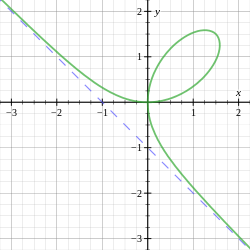# Folium of DescartesThe folium of Descartes (green) with asymptote (blue).

In geometry, the folium of Descartes is an algebraic curve defined by the equation.

It forms a loop in the first quadrant with a double point at the origin and asymptote.

It is symmetrical about.

The name comes from the Latin word folium which means "leaf".

The curve was featured, along with a portrait of Descartes, on an Albanian stamp in 1966.

## History

The curve was first proposed by Descartes in 1638. Its claim to fame lies in an incident in the development of calculus. Descartes challenged Fermat to find the tangent line to the curve at an arbitrary point since Fermat had recently discovered a method for finding tangent lines. Fermat solved the problem easily, something Descartes was unable to do. Since the invention of calculus, the slope of the tangent line can be found easily using implicit differentiation.

## Graphing the curve

Since the equation is degree 3 in both x and y, and does not factor, it is difficult to solve for one of the variables.

However, the equation in polar coordinates is:which can be plotted easily.

Another technique is to write y = px and solve for x and y in terms of p. This yields the rational parametric equations:.

We can see that the parameter is related to the position on the curve as follows:

• p < -1 corresponds to x>0, y<0: the right, lower, "wing".
• -1 < p < 0 corresponds to x<0, y>0: the left, upper "wing".
• p > 0 corresponds to x>0, y>0: the loop of the curve.

Another way of plotting the function can be derived from symmetry over y = x. The symmetry can be seen directly from its equation (x and y can be interchanged). By applying rotation of 45° CW for example, one can plot the function symmetric over rotated x axis.

This operation is equivalent to a substitution:and yieldsPlotting in the cartesian system of (u,v) gives the folium rotated by 45° and therefore symmetric by u axis.

## Relationship to the trisectrix of MacLaurin

The folium of Descartes is related to the trisectrix of Maclaurin by affine transformation. To see this, start with the equation,

and change variables to find the equation in a coordinate system rotated 45 degrees. This amounts to setting. In theplane the equation is.

If we stretch the curve in thedirection by a factor ofthis becomeswhich is the equation of the trisectrix of Maclaurin.

1. Simmons, p. 101
2. "DiffGeom3: Parametrized curves and algebraic curves". N J Wildberger, University of New South Wales. Retrieved 5 September 2013.
• J. Dennis Lawrence: A catalog of special plane curves, 1972, Dover Publications. ISBN 0-486-60288-5, pp. 106–108
• George F. Simmons: Calculus Gems: Brief Lives and Memorable Mathematics, New York 1992, McGraw-Hill, xiv,355. ISBN 0-07-057566-5; new edition 2007, The Mathematical Association of America (MAA)Wikimedia Commons has media related to Folium of Descartes.